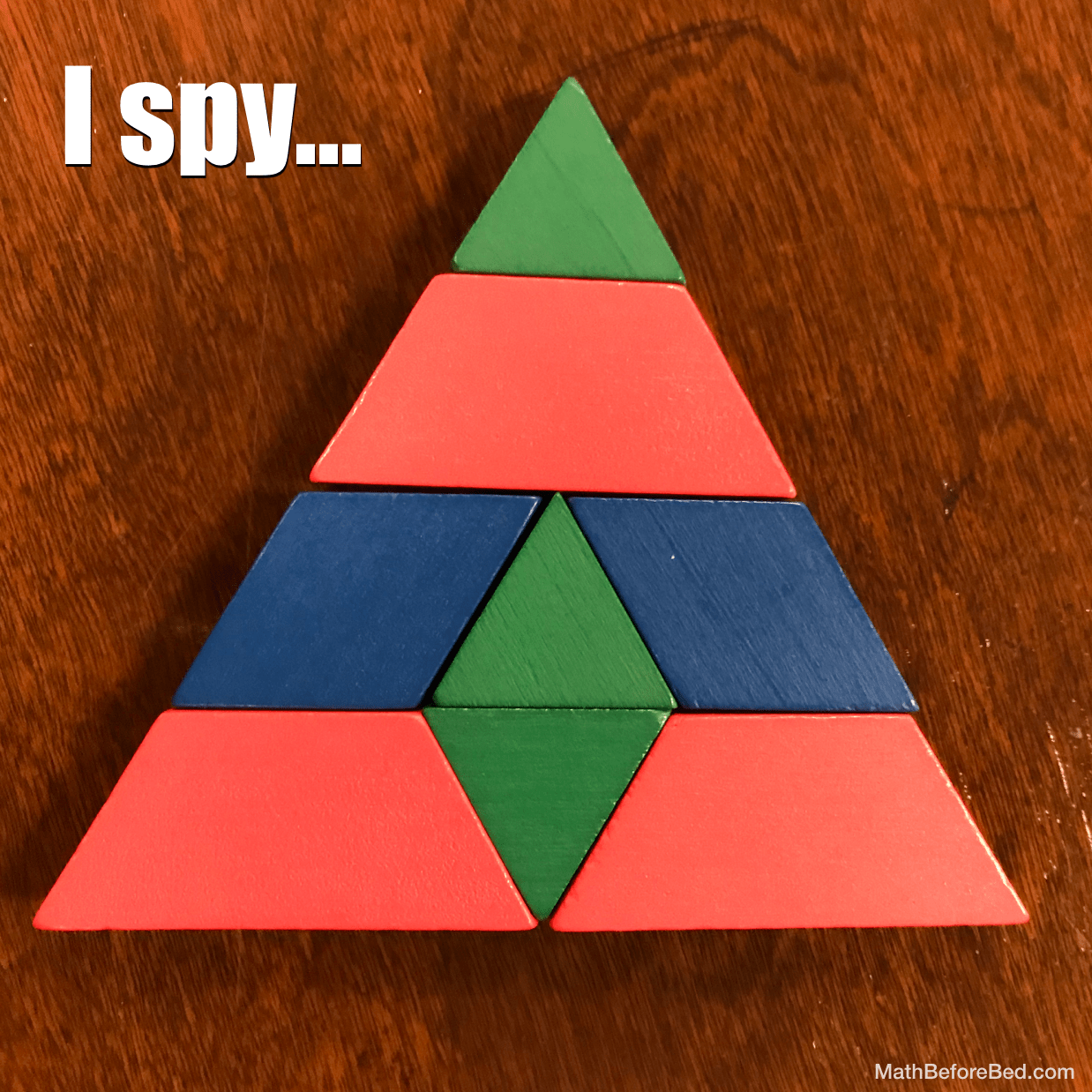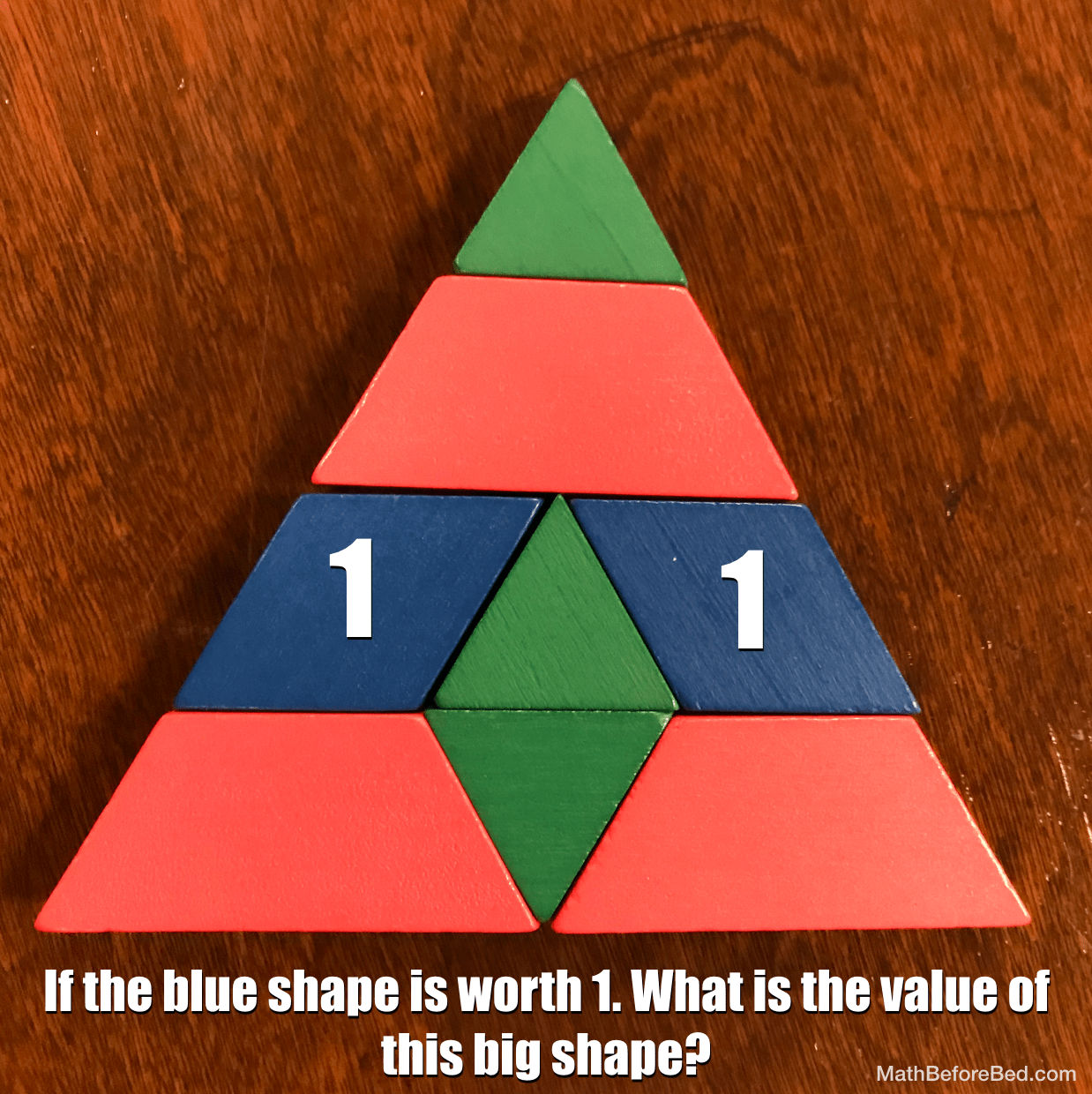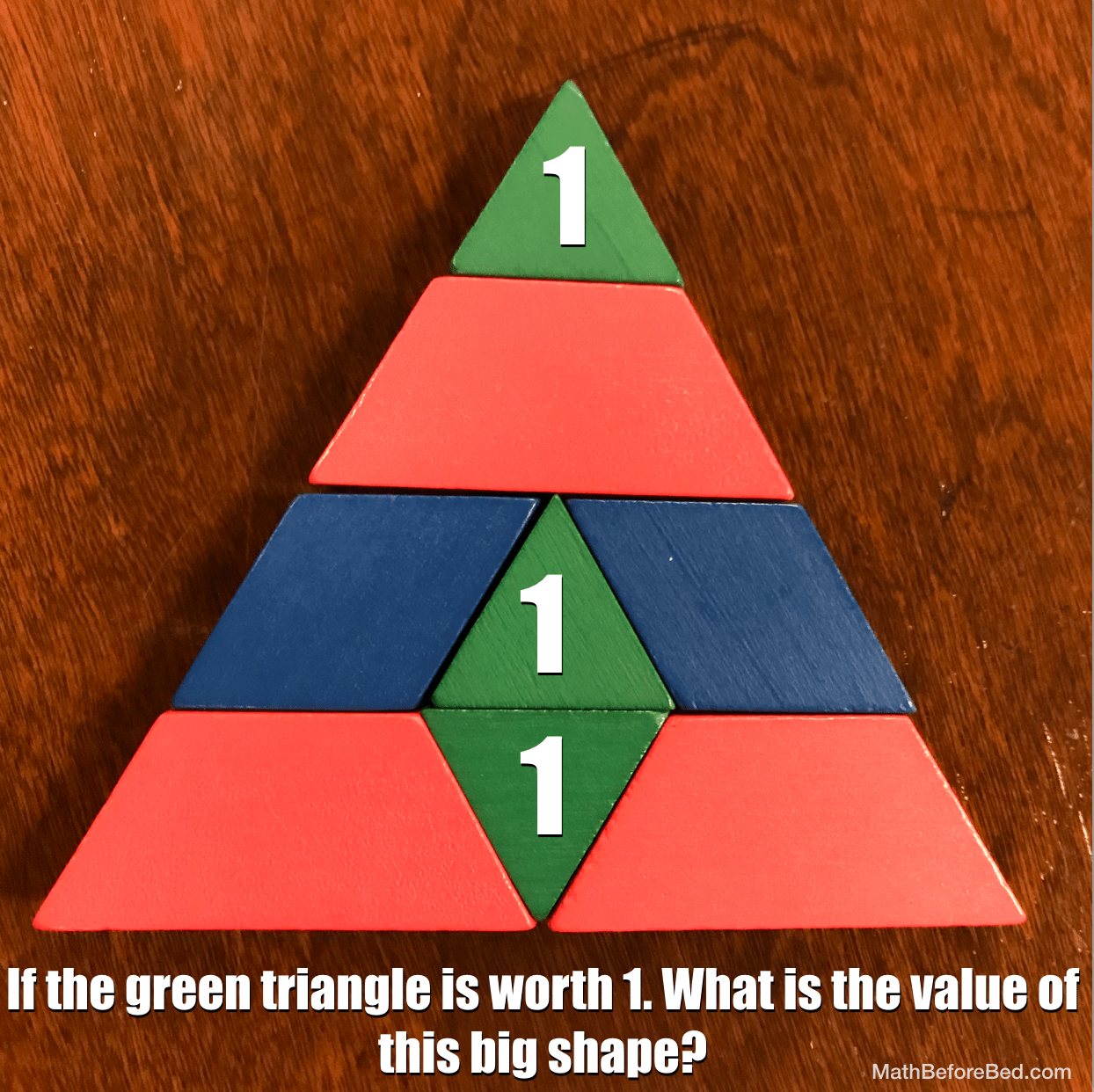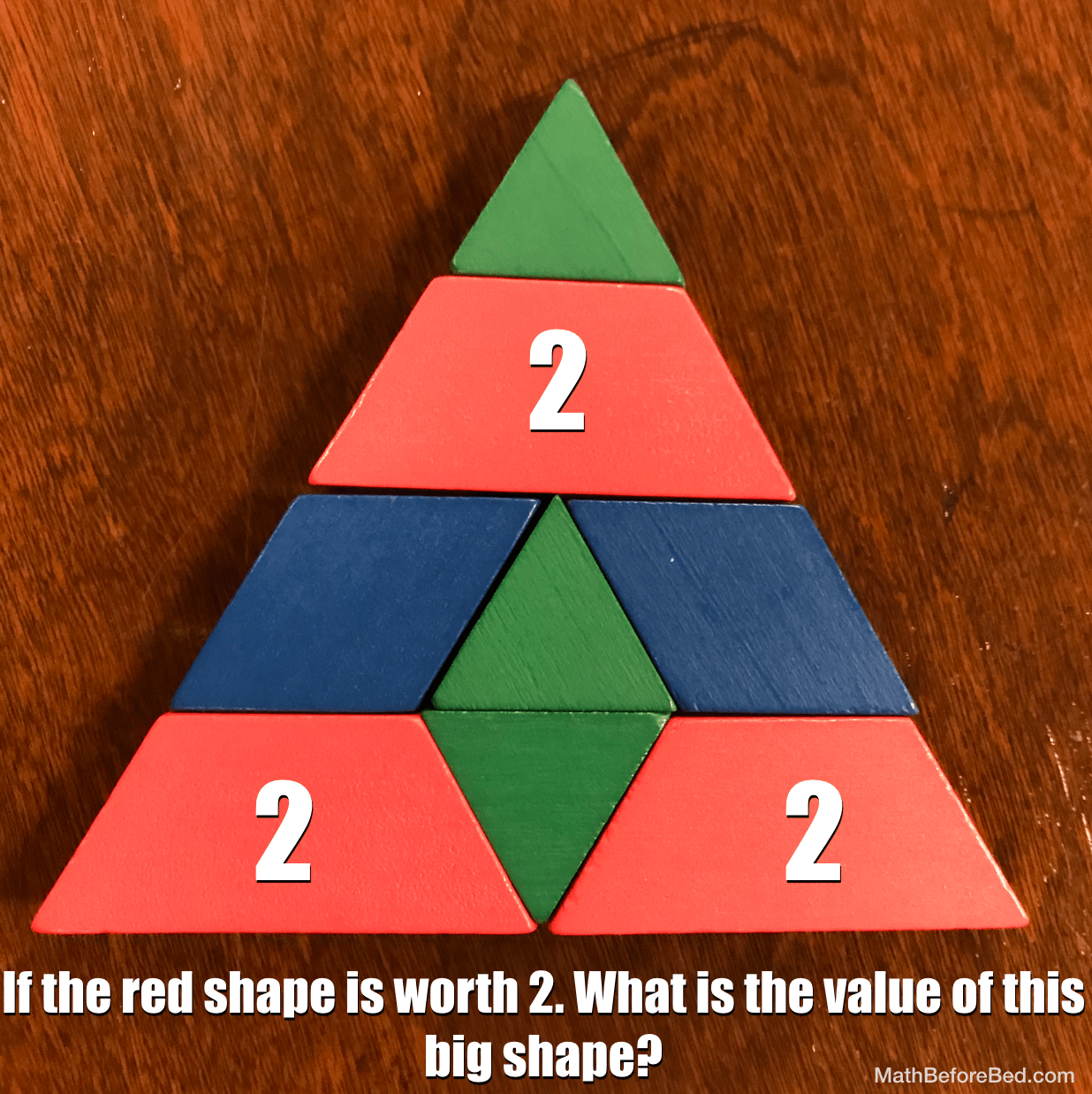# I Spy…Ask your students to look and share what they see. Potential discussion topics: How many shapes? How many of each colour? What is the perimeter? What fractions can you see?

Extensions:## 11 thoughts on “I Spy…”

1.Jeff Baumann says:

1+1+1=3

2.ruvel fagura says:

RED WORTH:1+1+1=3
BLUE WORTH:1+1=2
GREEN WORTH:1+1+1=3
RED WROTH:2+2+2=6

3.abigail says:

i spy 3 pink shapes

4.Oliver kahler says:

A triangle

5.Esther says:

blue 1+1=2
green 1+1+1=3
red 1+1+1=3
red 2+2+2=6

6.Cameron says:

I spy:
3 Triangles
1 diamond

Blue 1+1=2
Green 1+1+1=3
Red 1+1+1=3
Red 2+2+2=6

7.Cameron says:

Correction:
I spy….4 triangles

8.Marquee says:

Green 1+ 2 = 3
red 1+1+1= 3
Blue 1+1=2
Red 2+1=3

9.Edward. says:

I spy:shapes that are triangles,trapezoids.
I spy: 16 triangles.
I spy: the value 5 for the big shape ,when the red shape value 1.
I spy the value 10 for the big shape,when the red shape value 2.

10.Louisa Yorke says:

i spy:
3 triangles
1 diamond
i see:
3 green colors
3 red
2 blue
1+1=2 (blue)
1+1+1=3 (green)
1+1+1=3 (red)
2+2+2=6 (red)

11.SUDARON says:

GREEN WORTH:1+1+1=3
BLUE WORTH:1+1=2
RED WORTH:1+1+1=3
RED WORTH:2+2+2=6